# GMAT Math : DSQ: Calculating whether lines are perpendicular

## Example Questions

### Example Question #1 : Geometry

Data Sufficiency Question

Is Line A perpendicular to the following line?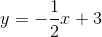Statement 1: The slope of Line A is 3.

Statement 2: Line A passes through the point (2,3).

Statement 1 alone is sufficient, but Statement 2 alone is not sufficient to answer the question.

Both statements taken together are sufficient to answer the question, but neither statement alone is sufficient.

Statement 2 alone is sufficient, but Statement 1 alone is not sufficient to answer the question.

Each statement alone is sufficient.

Statements 1 and 2 together are not sufficient, and additional data is needed to answer the question.

Statement 1 alone is sufficient, but Statement 2 alone is not sufficient to answer the question.

Explanation:

To determine if two lines are perpendicular, only the slope needs to be considered. The slopes of perpendicular lines are the negative reciprocals of each other. Knowing a single point on the line is not sufficient, as an infinite number of lines can pass through and individual point.

### Example Question #2 : Geometry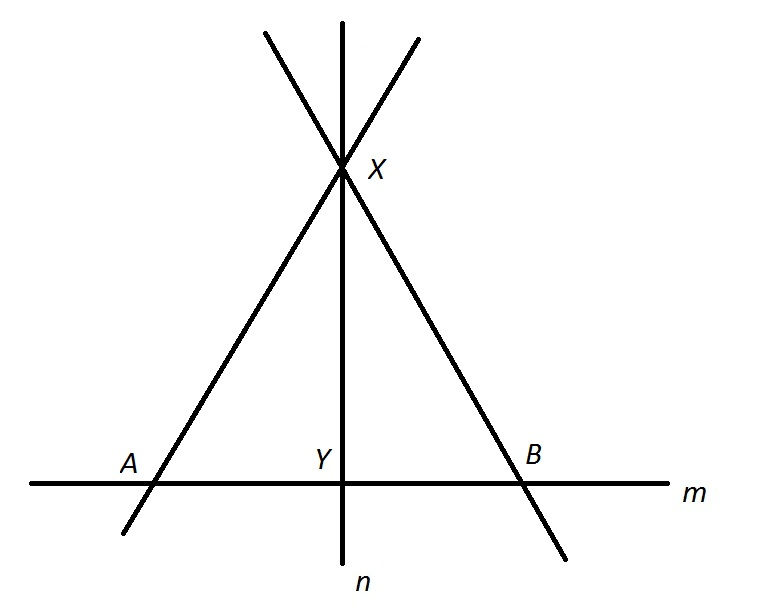Refer to the above figure. True or false: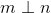Statement 1: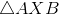is equilateral.

Statement 2: Linebisects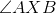.

Statement 1 ALONE is sufficient to answer the question, but Statement 2 ALONE is NOT sufficient to answer the question.

BOTH statements TOGETHER are insufficient to answer the question.

EITHER statement ALONE is sufficient to answer the question.

BOTH statements TOGETHER are sufficient to answer the question, but NEITHER statement ALONE is sufficient to answer the question.

Statement 2 ALONE is sufficient to answer the question, but Statement 1 ALONE is NOT sufficient to answer the question.

BOTH statements TOGETHER are sufficient to answer the question, but NEITHER statement ALONE is sufficient to answer the question.

Explanation:

Statement 1 alone establishes nothing about the anglemakes with, as it is not part of the triangle. Statement 2 alone only establishes that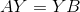.

Assume both statements are true. Then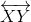is an altitude of an equilateral triangle, making it - and- perpendicular with the base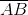- and.

### Example Question #1 : Geometry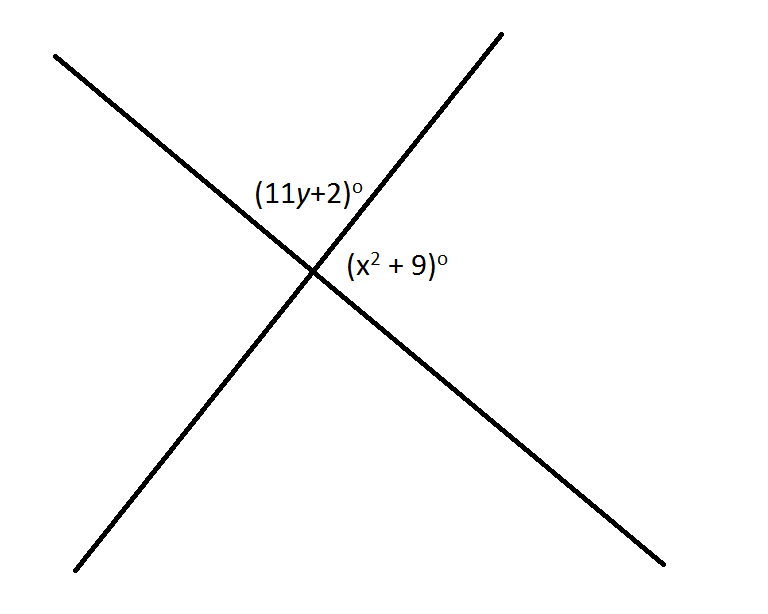Statement 1:

Refer to the above figure. Are the lines perpendicular?

Statement 1: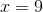Statement 2: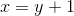Statement 1 ALONE is sufficient to answer the question, but Statement 2 ALONE is NOT sufficient to answer the question.

Statement 2 ALONE is sufficient to answer the question, but Statement 1 ALONE is NOT sufficient to answer the question.

BOTH statements TOGETHER are sufficient to answer the question, but NEITHER statement ALONE is sufficient to answer the question.

EITHER statement ALONE is sufficient to answer the question.

BOTH statements TOGETHER are insufficient to answer the question.

EITHER statement ALONE is sufficient to answer the question.

Explanation:

Assume Statement 1 alone. The measure of one of the angles formed is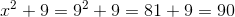degrees.

Assume Statement 2 alone.

By substituting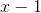for, one angle measure becomes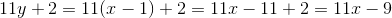The marked angles are a linear pair and thus their angle measures add up to 180 degrees; therefore, we can set up an equation: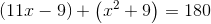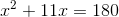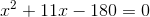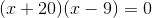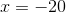oryields illegal angle measures - for example,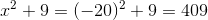yields angle measuresfor both angles; the angles are right and the lines are perpendicular.

### Example Question #1 : Geometry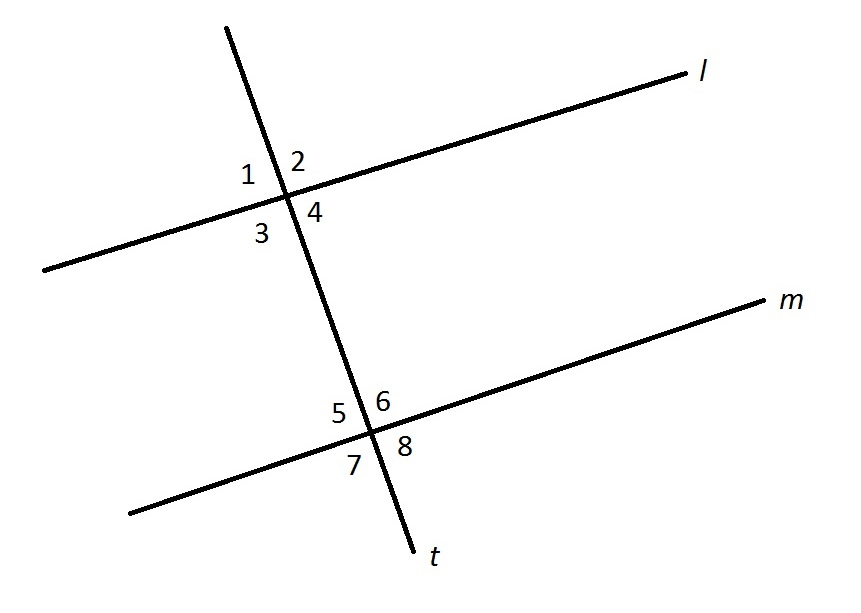Refer to the above figure.

True or false: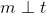Statement 1: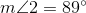Statement 2: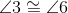EITHER statement ALONE is sufficient to answer the question.

Statement 2 ALONE is sufficient to answer the question, but Statement 1 ALONE is NOT sufficient to answer the question.

BOTH statements TOGETHER are insufficient to answer the question.

Statement 1 ALONE is sufficient to answer the question, but Statement 2 ALONE is NOT sufficient to answer the question.

BOTH statements TOGETHER are sufficient to answer the question, but NEITHER statement ALONE is sufficient to answer the question.

BOTH statements TOGETHER are sufficient to answer the question, but NEITHER statement ALONE is sufficient to answer the question.

Explanation:

Statement 1 alone establishes by definition that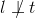, but does not establish any relationship betweenand.

By Statement 2 alone, since alternating interior angles are congruent,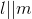, but no conclusion can be drawn about the relationship of, since the actual measures of the angles are not given.

Assume both statements are true. By Statement 2,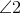and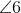are corresponding angles formed by a transversal across parallel lines, so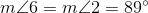is not a right angle, so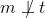.

### Example Question #5 : GeometryRefer to the above figure. True or false:Statement 1: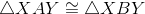Statement 2: Linebisects.

EITHER statement ALONE is sufficient to answer the question.

BOTH statements TOGETHER are insufficient to answer the question.

Statement 2 ALONE is sufficient to answer the question, but Statement 1 ALONE is NOT sufficient to answer the question.

Statement 1 ALONE is sufficient to answer the question, but Statement 2 ALONE is NOT sufficient to answer the question.

BOTH statements TOGETHER are sufficient to answer the question, but NEITHER statement ALONE is sufficient to answer the question.

Statement 1 ALONE is sufficient to answer the question, but Statement 2 ALONE is NOT sufficient to answer the question.

Explanation:

Assume Statement 1 alone. Then, as a consequence of congruence,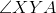and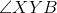are congruent. They form a linear pair of angles, so they are also supplementary. Two angles that are both congruent and supplementary must be right angles, so.

Assume Statement 2 alone. Then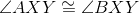, but without any other information about the angles that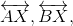ormake with, it cannot be determined whetheror not.

### Example Question #6 : Geometry

The equations of two lines are: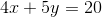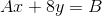Are these lines perpendicular?

Statement 1: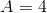Statement 2: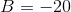Statement 2 ALONE is sufficient to answer the question, but Statement 1 ALONE is not sufficient to answer the question.

Statement 1 ALONE is sufficient to answer the question, but Statement 2 ALONE is not sufficient to answer the question.

BOTH statements TOGETHER are insufficient to answer the question.

EITHER  statement ALONE is sufficient to answer the question.

BOTH statements TOGETHER are sufficient to answer the question, but NEITHER statement ALONE is sufficient to answer the question.

Statement 1 ALONE is sufficient to answer the question, but Statement 2 ALONE is not sufficient to answer the question.

Explanation:

The lines of the two equations must have slopes that are the opposites of each others reciprocals.

Write each equation in slope-intercept form: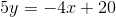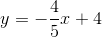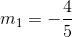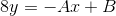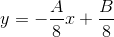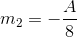As can be seen, knowing the value ofis necessary and sufficient to answer the question. The value ofis irrelevant.

The answer is that Statement 1 alone is sufficient to answer the question, but Statement 2 alone is not sufficient to answer the question.

Tired of practice problems?

Try live online GMAT prep today.